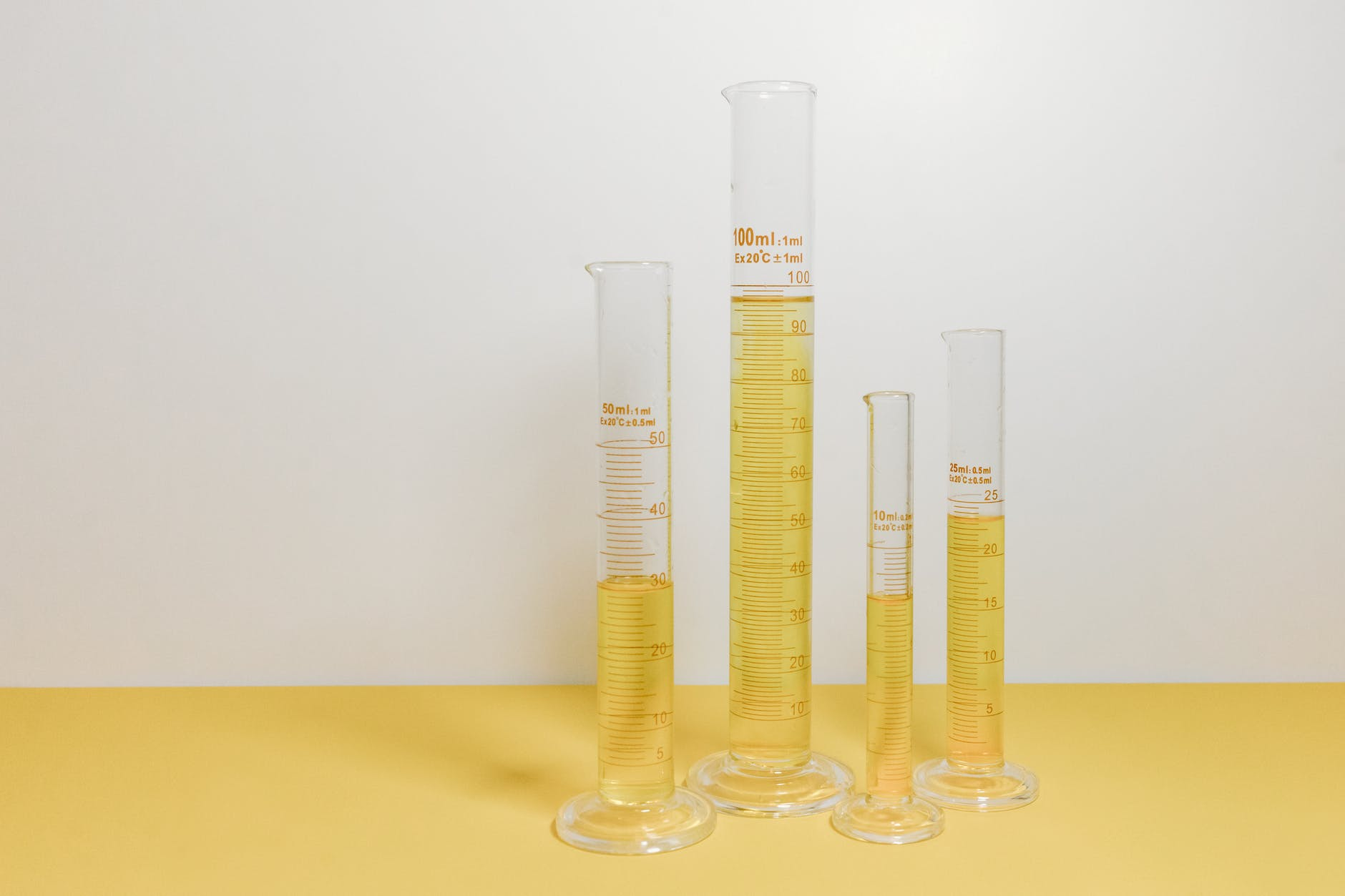# Solutes, Solvents, & Molality

A solution in chemistry is a homogenous mixture of two or more substances. The substances that are found in a solution can further be broken down into two types, and these are solute and solvent. The solute is the substance(s) dissolved into a solution. A solvent is a substance into which a solute is dissolved. In other words, solutes generally disappear into solvents. An example would be pouring salt into water. The salt is the solute, and the water is the solvent, and it appears that the salt disappears when added to water.

There is a limit to how much solute can be dissolved into a solvent. The term for this is solubility. Solubility varies from substance to substance but as an example, salt has a solubility of 35.9 grams per 100 grams of water. This means that you can dissolve 35.9 grams of salt in 100 grams of water. Any more salt, and there will be no more dissolving. The technical term when a solute can no longer dissolve in a solution is saturation, and the solution is now saturated.

Solubility is also affected by temperature. For a gas, the solubility increase as the temperature is lowered. However, the solubility of a gas increases with an increase in pressure. For solids, solubility actually increases with temperature.

Concentration

The concentration measures the amount of substance in a given volume. Concentration is measured by a unit called molarity. Molarity is the proportion of the moles of solute to the liters of solution. For example, suppose I have 150 grams of calcium nitrate, and I dissolve this into 1 liter of water. In that case, I can calculate the molarity as follows.

1. Determine the amu of calcium nitrate
1. This is calculated by finding the number of amu, which in this case is 164.10 amu
2. Convert the amu to moles
1. This is done by placing the original grams as the numerator of a fraction and the amu as the denominator, which is
2. 150/164.10 = 0.9141 moles
3. Use the ratio
1. Our answer is simple it is moles to solution as shown below
2. 0.9141 moles / 1 liter = 0.9141 M

Freezing and Boiling

A final point to mention is a term called freezing point depression. This involves mixing solutes and solutions that can change the freezing point of the substance. What is taking place is that when a solute is added to a solution, it now requires more energy to freeze the new substance. This is why salt is thrown on roads during icy days. The salt lowers the temperature at which ice can form, thus making the roads safer. However, there is a limit, and if it becomes cold enough, the salt will no longer have the desired effect.

Another factor involves the boiling point. Solutes increase the temperature that is needed for boiling to occur.

Conclusion

Solutes and solvents are among many terms used in chemistry to define the behavior of substances in a certain context. It is amazing how complex the world is and how there is always so much more that can be learned in various knowledge domains.